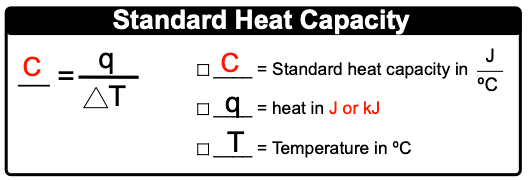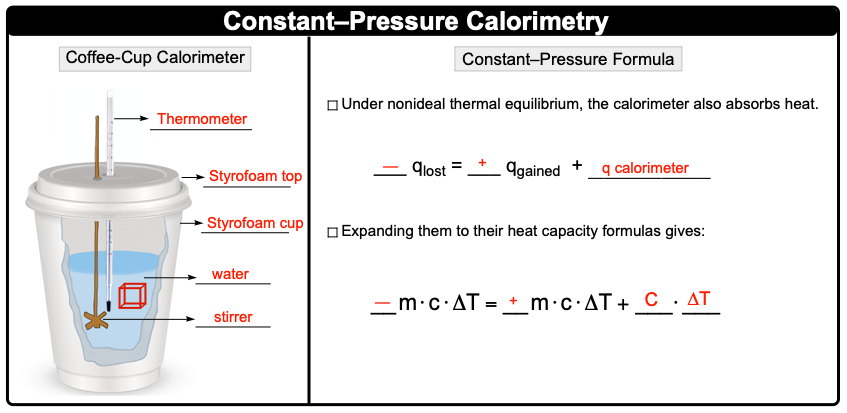Clutch Prep is now a part of Pearson
Ch.6 - Thermochemistry WorksheetSee all chapters

# Constant-Pressure Calorimetry

See all sections
Sections
Nature of Energy
Kinetic & Potential Energy
First Law of Thermodynamics
Internal Energy
Endothermic & Exothermic Reactions
Heat Capacity
Constant-Pressure Calorimetry
Constant-Volume Calorimetry
Thermal Equilibrium
Thermochemical Equations
Formation Equations
Enthalpy of Formation
Hess's Law
Enthalpy (IGNORE)

Constant-Pressure Calorimetry uses a coffee cup calorimeter to determine the heat transfers occurring in a liquid solution.

###### Constant Pressure Calorimetry

Concept #1: Constant-Pressure CalorimetryExample #1: What is the standard heat capacity (in kJ/ºC) of a calorimeter that absorbs 87.0 J as its temperature goes from 33 ºC to 38.1 ºC?

Concept #2: Coffee Cup CalorimeterExample #2: When 60.0 g of lead at 68.3 ºC is poured into 90.0 g H2O at 30.0 ºC within a coffee-cup calorimeter, the temperature increases to 48.0 ºC. Based on this information, what is the heat capacity of the calorimeter? The specific heat of lead and water are 0.128 J/g ∙ ºC and 4.184 J/g ∙ ºC respectively.

Practice: A 115.6 g piece of copper metal at 182.5 ºC is placed into 120.0 mL of methylene chloride at 31.0 ºC within a coffee-cup calorimeter. If the final temperature of the solution is 50.3 ºC, what is the specific heat of methylene chloride? Assume the calorimeter absorbs a negligible amount of heat. The specific heat of copper is 4.184 J/g ∙ ºC and the density of methylene chloride is 1.33 g/cm3.

Practice: You place 75.0 mL of 0.100 M NaOH in a calorimeter at 25.00 ºC and carefully add 55.0 mL of 0.200 M HNO3, also at 25.00 ºC. After stirring, the final temperature is 53.35 ºC. Calculate the enthalpy ∆Hrxn (in J/mol) for the formation of water. (Specific heat capacity, Cs, and density of the solution: 4.184 J/g∙K and 1.00 g/mL).